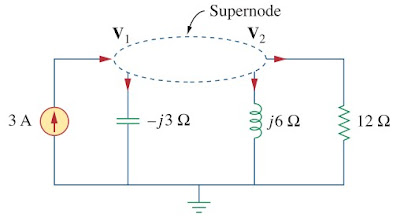omG8HI9LSKFW6lhCj8prTs0Z6lUhdOT9Jhi1Sf4m

# How to Use Nodal Analysis for AC Circuit Analysis

The basic of nodal analysis of ac circuit steady state condition is Kirchhoff's current law. Since KCL is valid for phasors, we can analyze ac circuit by nodal analysis.

## Nodal Analysis of AC Circuit

You will not find the basic theory of the nodal analysis here. We will only cover the step-by-step analyzing the ac circuit using this technique. If you have not learned about the its basic principle, make sure to read Nodal Analysis first.
The following nodal analysis ac circuit examples illustrate this.

Find the ix in the circuit of Figure.(1) using nodal analysis.Figure 1. Example of nodal ac circuit analysis
Solution :
We first convert the circuit to the frequency domain :
Hence, the frequency domain equivalent circuit is as drawn in Figure.(2).Figure 2. Frequency domain equivalent of the circuit in Figure.(1)
Applying KCL at node 1,
or(1.1)
At node 2,
But Ix = V1/-j2.5. Substituting this gives
By simplifying, we obtain(1.2)
Equations.(1.1) and (1.2) can be rewrite in matrix form to
We get the determinants as
The current Ix is given by
Transforming this to the time domain,

## Supernode Analysis of AC Circuit

Next we will analyze an ac circuit containing a supernode in the circuit. Like before, we will not cover the basic principle of supernode used in ac circuit. You need to read Supernode Analysis first in order to understand the following example.

Compute V1 and V2 in the circuit of Figure.(3).Figure 3. Example of supernode ac circuit analysis
Solution :
Nodes 1 and 2 form a supernode as drawn in Figure.(4).Figure 4. Supernode in the circuit
Applying KCL at the supernode gives
or(2.1)
But a voltage source is connected between nodes 1 and 2, so that(2.2)
Substituting Equations.(2.2) to (2.1) results
From Equation.(2.2),

Untuk Bahasa Indonesia baca Analisis Node Rangkaian AC
Related Posts
SHARE